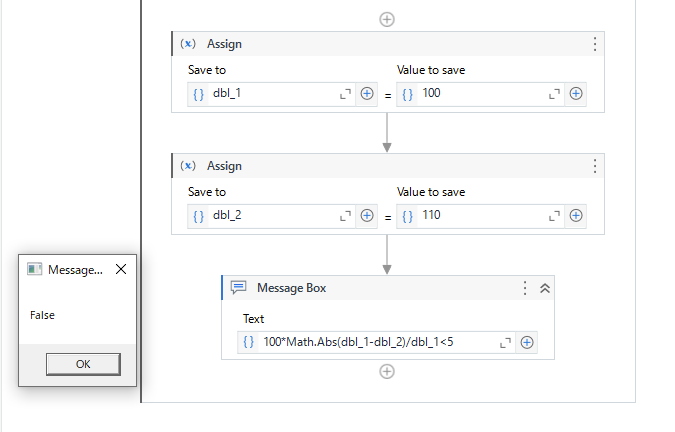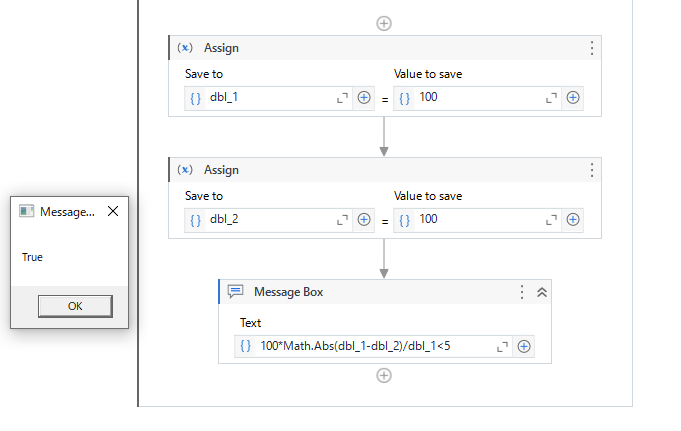# Compare two number with tolerance in %

Hello,
i need to compare two double variable with percentage tolerance.

Like

dbl_1 = 100.00
dbl_2 = 110.00
tolerance = 5%

how can i understand if the difference is > of the tolerance?

Thanks a lot

you can use the same condition how you calculate

val = ((dbl_2 - dbl_1)/dbl_1)*100
maxVal = 5
If ( val < maxVal)
acceptable value
Else
Not acceptable

1 Like

Great thank you

Hi,``````100*Math.Abs(dbl_1-dbl_2)/dbl_1<5
``````

Regards,

1 Like

thanks this is perfect!

i’m sorry bot it’s not perfect.
because with this solution if the variables containing the same number your solution give me a false answer.
I want if the number are the same give me a true answer.

thanks

Hi,

Can you elaborate? If both input value are same, this returns true as the following.Regards,